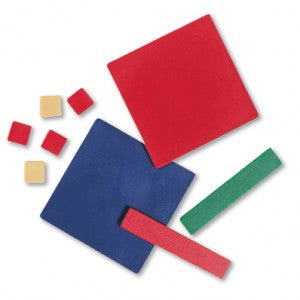Having fun. Growing minds.# Algebra Tiles Plastic Set 32pc

R 60.00Algebra tile manipulatives allow students to better understand algebraic thinking and algebra concepts. Algebra tiles are an excellent tool for students to solve algebra problems and use for algebra practice.
• Using algebra tiles provide concrete models for students when learning to add, subtract, and multiply integers.
• Use Algebra tiles for modeling and simplifying algebraic expressions, distributive property, solving linear equations, and multiplying polynomials and binomials.
Each student set includes 32 plastic pieces which represent +/-. The Algebra tile 32 piece set includes, 4 - x² variables, 8 - xy variables, and 20 - y constants.

Also available as an Algebra Tiles™ Classroom Set

Ages 11+

Product Code: LER 7540